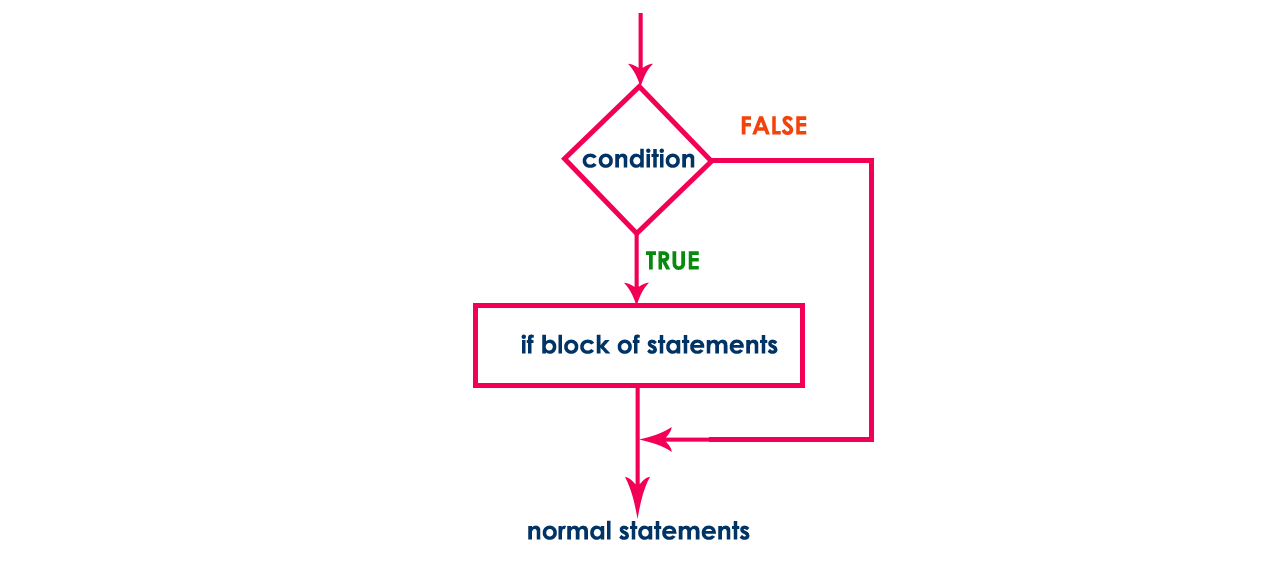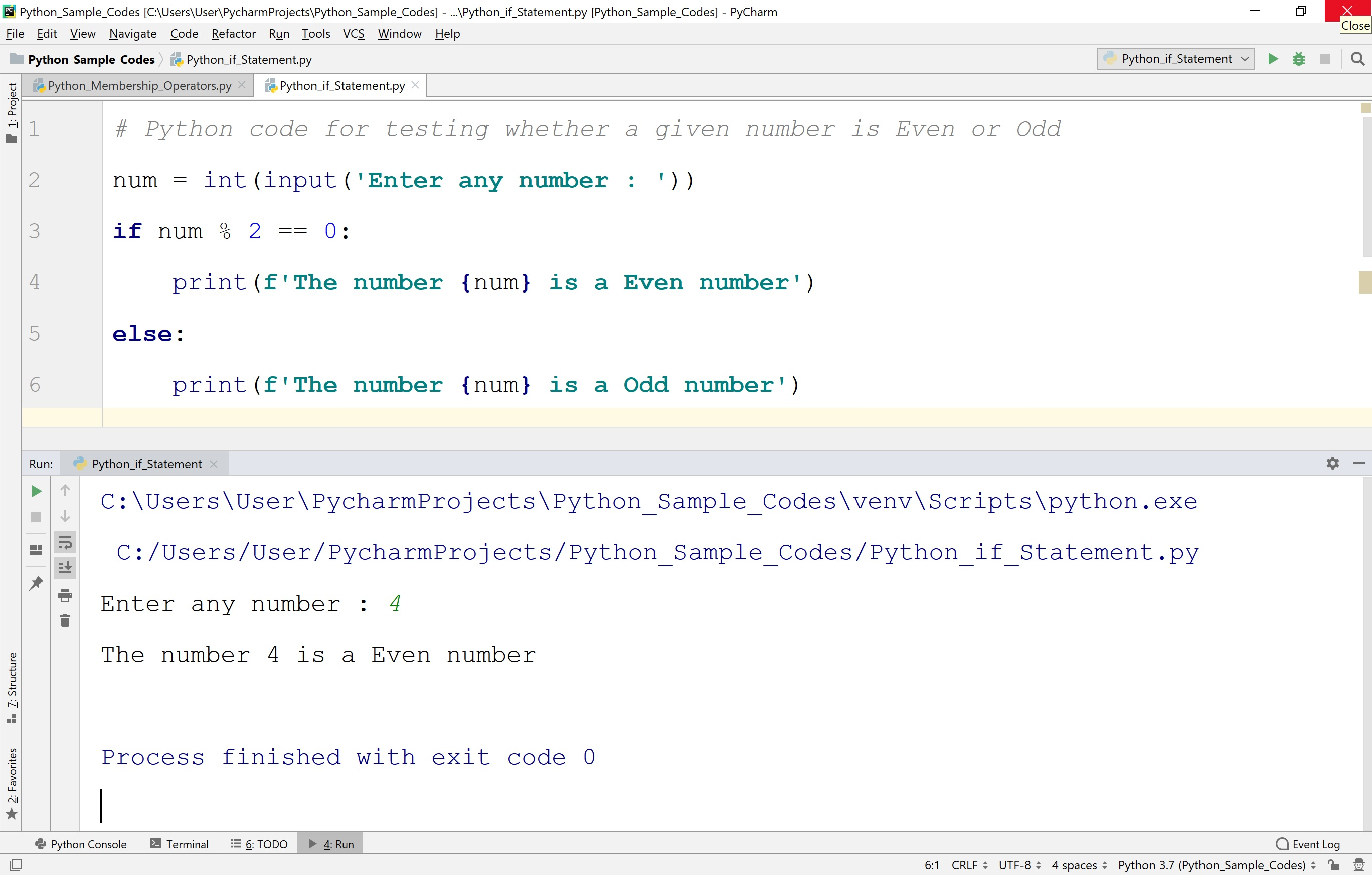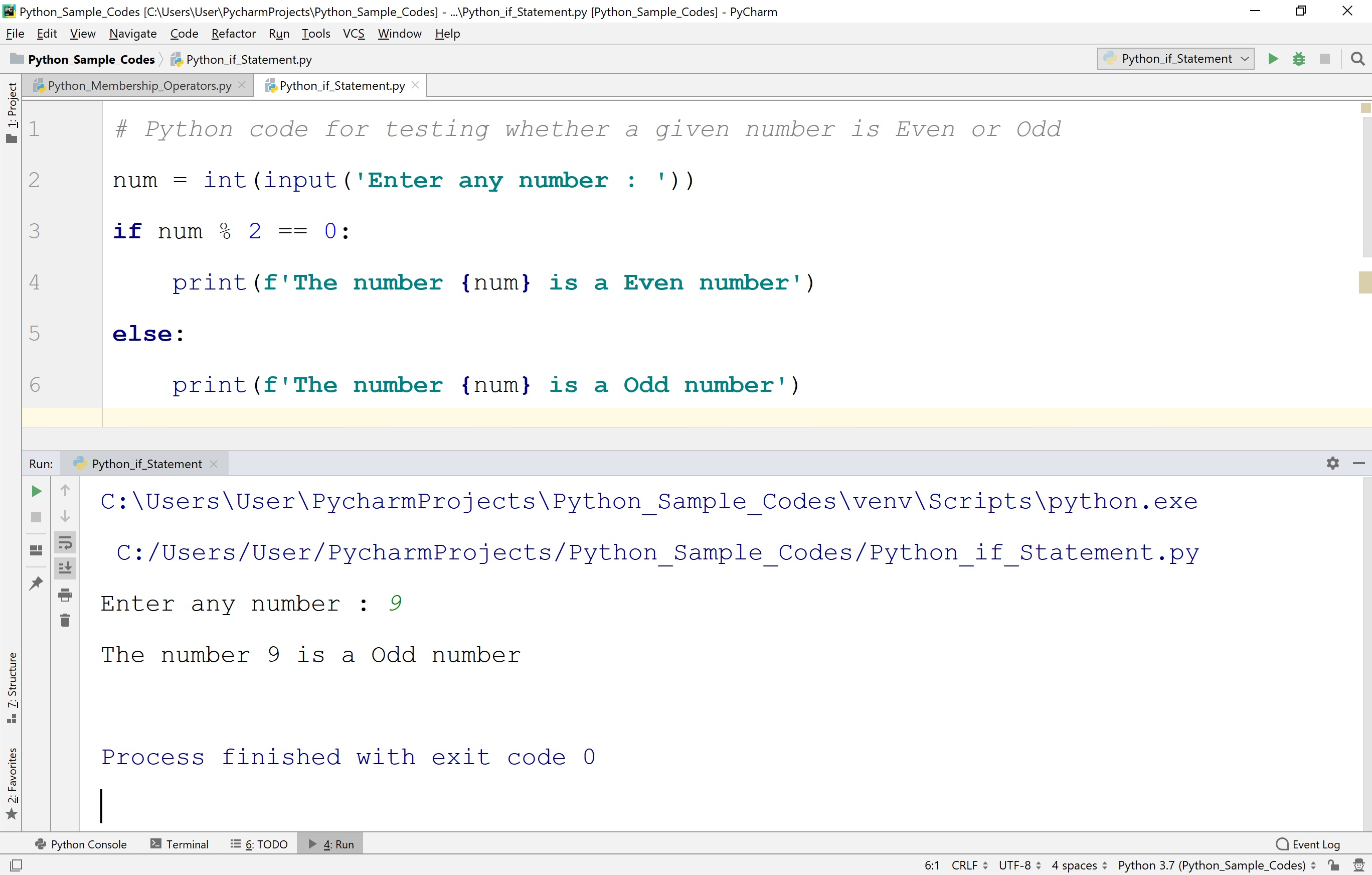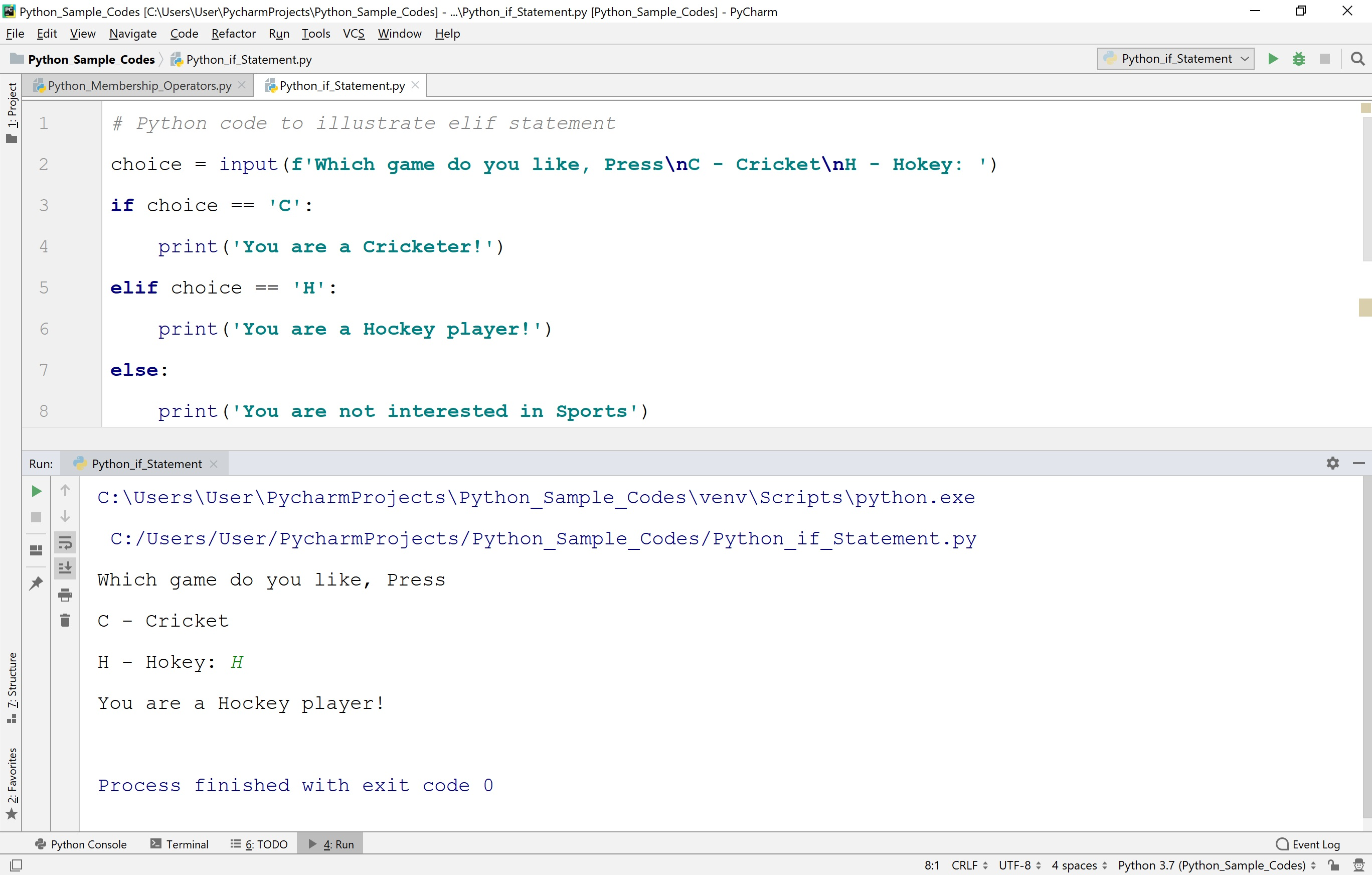The perfect place for easy learning...

×

# Python Selection Statements

In Python, the selection statements are also known as decision making statements or branching statements. The selection statements are used to select a part of the program to be executed based on a condition. Python provides the following selection statements.

• if statement
• if-else statement
• if-elif statement

## if statement in Python

In Python, we use the if statement to test a condition and decide the execution of a block of statements based on that condition result. The if statement checks, the given condition then decides the execution of a block of statements. If it is True, then the block of statements is executed and if it is False, then the block of statements is ignored. The execution flow of if statement is as follows.The general syntax of if statement in Python is as follows.

### Syntax

```if condition:
Statement_1
Statement_2
Statement_3
...```

When we define an if statement, the block of statements must be specified using indentation only. The indentation is a series of white-spaces. Here, the number of white-spaces is variable, but all statements must use the identical number of white-spaces. Let's look at the following example Python code.

# Python code to illustrate if statement

``````
num = int(input('Enter any number: '))
if (num % 5 == 0):
print(f'Given number {num} is divisible by 5')
print('This statement belongs to if statement')
print('This statement does not belongs to if statement')
```
```

When we run the above code, it produces the output as follows.When we enter a number which is not divisible by 5, then it produces the output as follows.In the above execution, the number 9 is not divisible by 5. So, the condition becomes False and the condition is evaluated to False. Then the if statement ignores the execution of its block of statements.

## if-else statement in Python

In Python, we use the if-else statement to test a condition and pick the execution of a block of statements out of two blocks based on that condition result. The if-else statement checks the given condition then decides which block of statements to be executed based on the condition result. If the condition is True, then the true block of statements is executed and if it is False, then the false block of statements is executed. The execution flow of if-else statement is as follows.The general syntax of if-else statement in Python is as follows.

### Syntax

```if condition:
Statement_1
Statement_2
Statement_3
...
else:
Statement_4
Statement_5
...```

In the above syntax, whenever the condition is True, the statements 1 2 and 3 are gets executed. And if the condition is False then the statements 4 and 5 are gets executed. Let's look at the following example of Python code.

# Python code to illustrate if-else statement

``````
# Python code for testing whether a given number is Even or Odd
num = int(input('Enter any number : '))
if num % 2 == 0:
print(f'The number {num} is a Even number')
else:
print(f'The number {num} is a Odd number')
```
```

When we run the above code, it produces the output as follows.## elif statement in Python

In Python, When we want to test multiple conditions we use elif statement.

The general syntax of if-elif-else statement in Python is as follows.

### Syntax

```if condition_1:
Statement_1
Statement_2
Statement_3
...
elif condition_2:
Statement_4
Statement_5
Statement_6
...
else:
Statement_7
Statement_8
...```

In the above syntax, whenever the condition_1 is True, the statements 1 2 and 3 are gets executed. If the condition_1 is False and condition_2 is True then the statements 4, 5, and 6 are gets executed. And if condition_1 nad Condition_2 both are False then the statements 7 and 8 are executed. Let's look at the following example of Python code.

# Python code to illustrate if-else statement

``````
# Python code to illustrate elif statement
choice = input(f'Which game do you like, Press\nC - Cricket\nH - Hokey: ')
if choice == 'C':
print('You are a Cricketer!')
elif choice == 'H':
print('You are a Hockey player!')
else:
print('You are not interested in Sports')
```
```

When we run the above code, it produces the output as follows.